# How to Calculate Relative Standard Deviation in Excel?

• Difficulty Level : Expert
• Last Updated : 01 May, 2021

Definition: Related standard deviation is also known as the relative percentage standard deviation form, the deviation measurement which tells us how different numbers are dispersed around the mean in a particular set of data. This format shows the percentage distribution of data.

If a relative standard deviation of the product is higher, that means that the numbers are very wide-ranging from the mean of that product. The RSD team sometimes needs certain data which is far removed from the Average RSD according to product requirement. Data that are well deviated from the RSD are taken into consideration in those cases.

In the event of the reverse situation, i.e. a lower relative default, the numbers are closer than the average and are also referred to as the coefficient of difference. In general, the idea of actual forecasts is given within the given data set.

### Uses of Relative Standard Deviation:

1. Relative standard deviation is used widely in the interpretation of statistical data relations in different segments.
2. This is one of the primary tools indicating whether the stock price is changing the growth of the company.
3. RSD is a sophisticated version of an analytical tool that assists the end-user in understanding trends, product demand, and customer preferences across various industries. RSD thus helps to detect the actual results of various options.

### Formula:

RSD(Relative Standard Deviation)=s×100 / x¯

where,

RSD = Relative standard deviation

s = Standard deviation

x¯ = Mean of the data.

Example 1: Calculate RSD when numbers: 8,20,40,60 and the standard deviation is 5.

First calculating mean(x)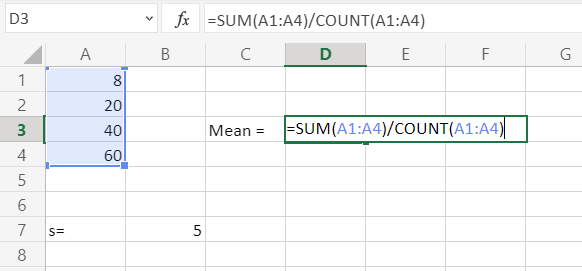Then calculating RSD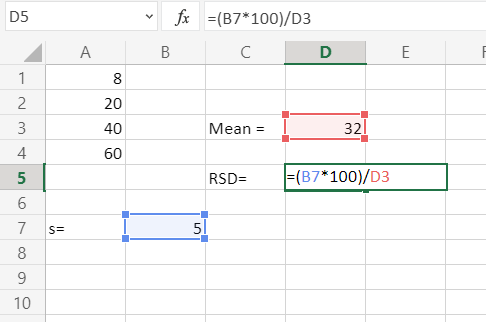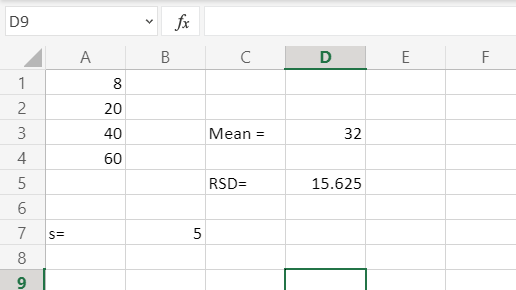Evample 2: Calculate RSD when numbers: 10,20,30,40,50 and the standard deviation is 10.

First calculating mean(x)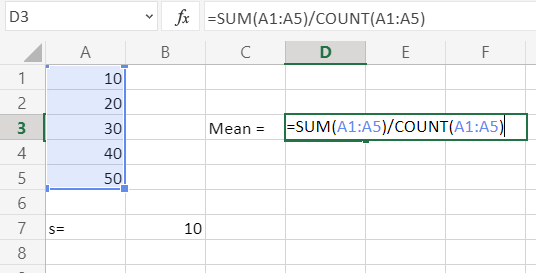Then calculating RSD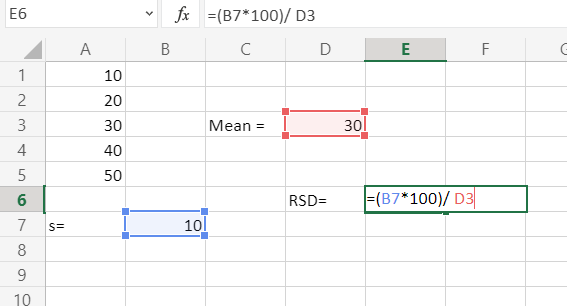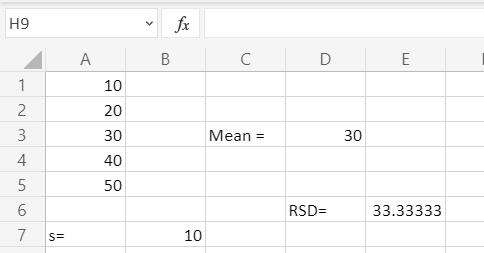My Personal Notes arrow_drop_up